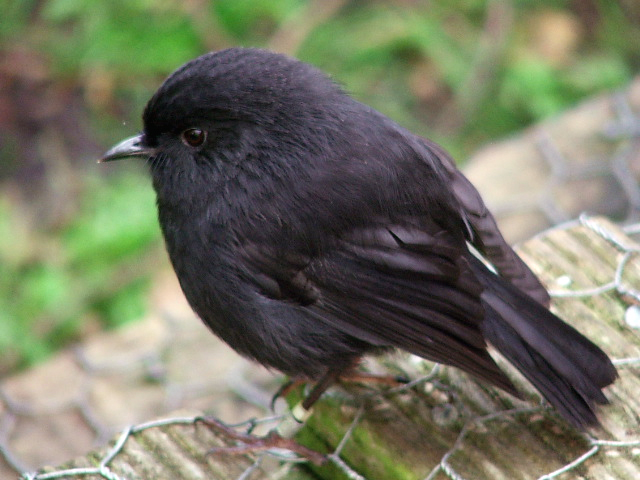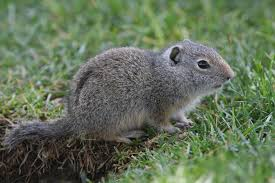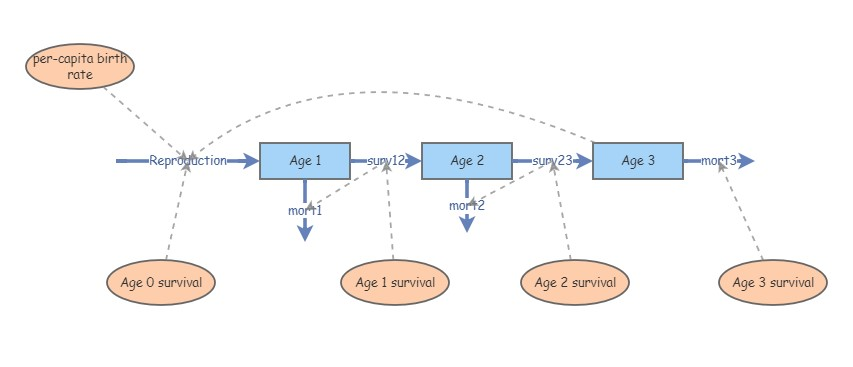In this lab we will have an opportunity to build more complex population models in InsightMaker. Among the concepts we will play around with are age structure, life tables, and age/stage matrices.

First let’s do some math!

## Mathematics of Age-structured populations

### Life tables

Let’s start with some definitions- population ecology, and life tables in particular, are full of terms and equations. We should at least be aware of the meaning of the major terms involved in life table analysis!

Cohort: A group of organisms of the same species that are born during the same year or the same breeding season

x: Age, or time elapsed since the birth date of the cohort (often in years).

S(x): Survivors, or Census size – the number of individuals in the study population who survive to time x.

D(x): Deaths – the number of age x individuals who die before reaching age x+1

Birth rate, b(x): Average number of offspring produced by an individual in age category x (per-capita birth rate for a given age). NOTE: this parameter is sometimes called m(x) for “maternity”

Survivorship, l(x): $$l(x) = \frac{S_x}{S_0} \qquad \text{(Eq. 1)}$$ , where $$S_x$$ is the number of survivors from the original cohort at year $$x$$

Survival rate, g(x): $$g(x) = \frac{S_{x+1}}{S_x} \qquad \text{(Eq. 2)}$$ , where $$S_x$$ is the number of survivors from the original cohort at year $$x$$ (per-capita survival rate at age x)

Lifetime reproductive potential: $$R_0 = \sum_{x=0}^k l(x)\cdot b(x) \qquad \text{(Eq. 3)}$$, where $$k$$ is the maximum possible age. Lifetime reproductive potential is the average number of female offspring produced per female over her entire lifetime.

Life Expectancy (for a newborn) can be approximated as: $$LE_0 = \frac{\sum_{x=0}^{k}S(x+1)+0.5*D(x)}{S(0)} \qquad \text{(Eq. 4)}$$

Q: why is the above estimate for life expectancy (LE) approximate and not exact? Is it likely to be biased or unbiased?

Generation time is defined as the Average parental age (at birth) for all the offspring produced by a cohort. This can be computed as: $$G = \frac{\sum_{x=0}^{k}l(x)\cdot b(x)\cdot x}{\sum_{x=0}^{k}l(x)\cdot b(x)} \qquad \text{(Eq. 5)}$$

Intrinsic rate of growth, $$r$$ is defined (to a first-order approximation) as: $$r = \frac{ln(R_0)}{G} \qquad \text{(Eq. 6)}$$

NOTE: the Gotelli book describes a more exact way to compute the intrinsic rate of growth from life tables, called the Euler method.

One more concept I want to introduce here is reproductive value, or V(x). This represents the expected future reproductive output of an individual of age x, discounted by the intrinsic rate of growth: $$V(x) = \frac{e^{rx}}{l_x} \cdot \sum_{y=x+1}^{k}e^{-ry}l_yb_y \qquad \text{(Eq. 7)}$$. Different individuals in a population tend to have different “value” in terms of contributing to future generations. Caswell (2001) said “The amount of future reproduction, the probability of surviving to realize it, and the time required for the offspring to be produced all enter into the reproductive value of an age-class”. Knowing something about the relative “value” of different individuals in a population can help managers decide which individuals to translocate, or to harvest, or cull from a population. Therefore, reproductive value is critical for applied population ecology! Reproductive Value also factors heavily in evolutionary studies and life history theory.

Q: Why is the reproductive value discounted by the population growth rate??

## Exercise 1: life table analysisLet’s imagine that we are following a cohort of reintroduced Chatham Island robins on a small island through time.

• First, we establish artificial nests and place 350 captive-laid eggs in them.
• All individuals are given a unique marking as soon as they hatch (hatching is 100% successful!). These markings are permanent and not affected by tag loss!
• We visit the island once per year, and count all the female individuals with tags who still exist in the population. We can assume that if the individual is alive then we will observe it (perfect detection!). If we do not see the individual we know with certainty that it is dead!
• We record the following numbers of robins over 5 years revisiting the island (starting with 350 at year 0): 50, 25, 10, 2, and 0.
• We record the following per-capita reproductive rates for each age: 0, 5.2, 5.5, 4.7, 3.3, and 0

You can load these data in this CSV file. It should look something like this:

x S(x) b(x)
0 350 0.0
1 50 5.2
2 25 5.5
3 10 4.7
4 2 3.3
5 0 0.0

### QUESTIONS, Exercise 1 (basic life tables):

1a. Plot the survivorship curve (survivorship over age) in two ways: (1) raw survivorship vs. age, and (2) log (base 10) transformed survivorship vs. age (this is the “correct” way!). Does this population most closely resemble type I, II, or III survivorship? You only need to include the log-tranformed figure in your submitted response. Why do you need to log-transform the survivorship values in order to properly classify this population as type I, II or III? [HINT: would you be able to distinguish type II and III survivorship curves if you didn’t log-transform the y axis?]

1b. What is the lifetime reproductive potential, $$R_0$$ for this population? Based on your estimate of $$R_0$$ is this population growing, declining or stable? What happens to $$R_0$$ if “age 1” individuals do not reproduce – that is, if b(1) is set to zero?

1c. What is the life expectancy from birth, $$LE$$, and generation time, $$G$$ for this population? What happens to $$G$$ if “age 1” individuals do not reproduce – that is, if b(1) is set to zero?

1d. What is the reproductive value($$V$$) for each age (x) in this population? Which age has the highest reproductive value? If you were to remove some of this population to translocate to another island (to start a new population), why might you select individuals at this age (that is, the age with highest reproductive value)?

1e. Are the vital rates (e.g., b(x) or g(x)) in this population Density-dependent? If so, explain your reasoning. If not, explain what additional information you would need to evaluate density-dependence in this population.

## Exercise 2: another life table analysisLet’s take the Uinta ground squirrel example from the Gotelli book. Take a minute to read the description in Gotelli (end of Ch. 3)…

This is real data from a long-term experimental study.

The first life table (on the left) represents a cohort of ground squirrels in a population at typical densities.

The second life table (right) represents a cohort of ground squirrels in a population at lower-than-average densities (many conspecifics removed prior to the start of the experiment).

Load up the Uinta ground squirrel life table data given to us by Gotelli (reproduced from Slade and Balph 1974).

### QUESTIONS, Exercise 2 (more advanced life table analysis):

2a. [for the Uinta squirrel life table] Compare the two life tables using the following major life-table metrics: $$R0$$, $$G$$, and $$r$$ (extra credit: compare the reproductive values $$V$$ for the squirrels at each age). What differences do you notice between these two life tables?

2b. [for the Uinta squirrel life table] Is generation time ($$G$$) an intrinsic trait of a species, or can it vary as a function of factors like forage quality? How could you test this in Excel? What l(x) or b(x) entries might change in the Excel spreadsheet if the population experienced a substantial improvement in forage quality? Try it- describe what you changed, and how (and if) it affected generation time.

2c. [return to the black robin example] Imagine you have the following initial population of black robins: 10 individuals of age 2, 10 individuals of age 3 and 10 individuals of age 4. How would you simulate the abundance of robins in each of the possible ages (age 0,1,2,3,4,and 5) next year? Try it, and show how you got your answer!

## Exercise 3: age-structured models in InsightMaker

Let’s build an age-structured model from scratch in InsightMaker. To do this, you can follow these steps:

1. Open a blank workspace and save it.

2. Make three new stocks: Age 1, Age 2 and Age 3.

3. Make a new flow (flow in) called Recruitment. This should represent new Age 1 individuals. We will ignore immigration for now, so new recruiment into the “Age 1” stock is the only way new individuals can be added to the population. We will assume that Age 2 and Age 3 are the only reproductively active ages. Therefore, draw links from Age 2 and Age 3 to Recruitment.

4. Make new flows from Age 1 to Age 2 and from Age 2 to Age 3, called Transition to Age 2 and Transition to Age 3, respectively. These flows represent growth, or advancement from Age 1 to Age 2 is dependent on the number of individuals in Age 1. Therefore, draw a link from Age 1 to Transition to Age 2. Do the same for Transition to Age 3.

5. Make three new flows representing, emerging respectively from each stock and called Age 1 mortality, Age 2 mortality, and Age 3 mortality. The total number of deaths is dependent on the numbers in each stock, so draw links from each stock to the respective mortality outflow.

Your new insight should look something like this:NOTE: you don’t need links from flows to connected stocks.

1. Parameterize your new age-structured population! 6a. For recruitment, make new variables called Recruitment rate, age 2 and Recruitment rate, age 3, representing the per-capita recruitment rate for age 2 and age 3, respectively. Draw links from these variables to Recruitment, and set these variables at 1.1 and 1.8, respectively. Click on the equation editor for Recruitment and set the equation appropriately (see questions below) 6b. For the transitions, make new variables representing the per-capita transition rates, called Transition rate, Age 1 to 2 and Transition rate, Age 2 to 3. Draw links to the appropriate flows, and set these rates at 0.4 and 0.3, respectively. NOTE: these transition rates could also be called “survival rates”
6c. For the mortality rates, note that all individuals in the Age 1 stock must either transition to Age 2 or die (mortality rates are 1 minus the corresponding transition rate). In addition, all individuals in the Age 3 stock must die- there is no Age 4 class! Now click on the equation editors for the mortality rates, and specify the mortality rates appropriately.

2. Explore the model- make sure you understand how it works!

### QUESTIONS, Exercise 3 (age-structured model in InsightMaker):

3a. What is the equation for Recruitment in the above model? Copy and paste your equation from InsightMaker. Now explain this equation in plain English!

3b. What is the equation for Age 1 Mortality in the above model? Copy and paste the equation from InsightMaker. Now explain this equation in plain English! What about Age 3 mortality?

3c. Initialize the population abundances (Age 1, Age2, and Age 3 initial abundances) so that the population consists of only individuals in the first (Age 1) age class (e.g., 100 individuals in Age 1, no individuals in the other age classes). How do the population dynamics at the beginning of the simulation differ from the dynamics at the end of the simulation?

3d. Can you tweak the initial abundances so that the population exhibits smooth exponential growth/decline for all three age classes? What is the Stable Age Distribution for this population? Does the stable age distribution change if you change the vital rates (recruitment rates, transition rates etc.)? When you are done, please return all population vital rates to their original specifications.

3e. Is this a growing or declining population (make sure you use the originally specified vital rates)? Imagine you could enact a predator-control program and reduce the mortality of Age 1 individuals. How much would you have to reduce mortality (i.e., increase the proportion transitioning from Age 1 to age 2) of this age class to make a growing population? What about Age 2 mortality – how much would you have to reduce mortality of this age class (increase the proportion transitioning from Age 2 and Age 3) to make a growing population?

## Exercise 4: more complex age-structured models in InsightMaker!

Try to implement the following model (adding onto the previous age-structured model in InsightMaker). If the total population (all three age classes combined!) exceeds 75 individuals, then recruitment rates drop to 25% of normal rates.

4a. Please provide your full InsightMaker model (copy URL in the appropriate place in WebCampus).

4b. Change the Age 1 mortality rate to 0.3 (i.e., change the survival/transition rate to 0.7). Run the simulation starting with 75 individuals, all in Age class 1. What happens? Is this a stochastic model (different results every time you run the model, even with the same initial conditions)? If not, why does the population fluctuate back and forth as if it had a random component?

##Checklist for Lab 3 completion

• Please type your responses into one Word document and submit using WebCampus!

Due Feb. 28 at midnight.

• Word document with short answers
• Exercise 1